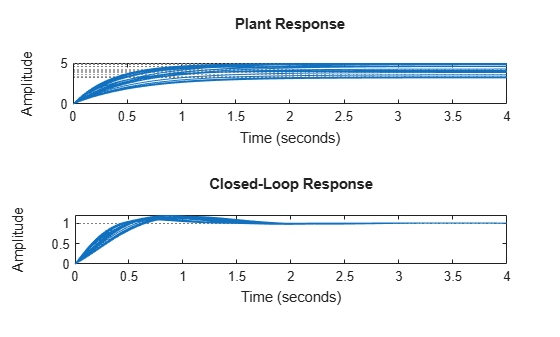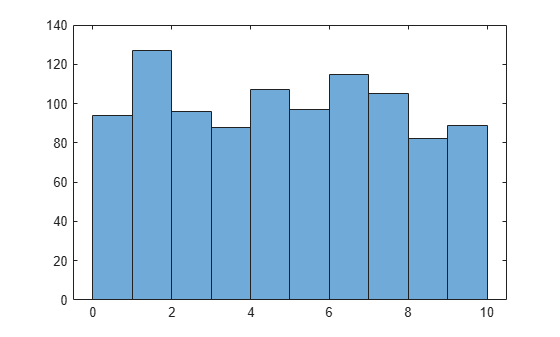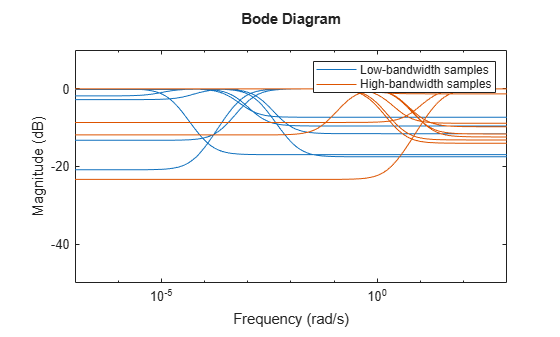# usample

Generate random samples of uncertain model or element

## Syntax

``B = usample(A,N)``
``B = usample(A,Names,N)``
``B = usample(A,Names1,N1,...,Namesk,Nk)``
``B = usample(___,wmax)``
``[B,SampleValues] = usample(___)``

## Description

Use `usample` to generate random samples of uncertain elements, such as `ureal` parameters, or models containing uncertain elements, such as `uss` models. Sampling replaces an uncertain element with a random specific value of the same type that falls in the range of the uncertain element. For instance, `usample` replaces a `ureal` parameter with random real scalar values within the parameter range. Similarly, `usample` replaces an `ultidyn` element with specified dynamics that fall within the specifications of the `ultidyn` element.

To generate random samples of uncertain variables in an Uncertain State Space block in a Simulink® model, see `usample`.

example

````B = usample(A,N)` substitutes `N` random samples of the uncertain elements in `A`, returning a certain (that is, not uncertain) array with the dimensions `[size(A) N]`. `A` can be:An individual uncertain element such as a `ureal` parameter or a `ultidyn` element. An uncertain model, such as a `uss` or `genss` model, that contains one or more uncertain elements.If you omit `N`, then `usample` returns a single sample.```

example

````B = usample(A,Names,N)` samples only the uncertain elements listed in `Names`. If `Names` does not include all the uncertain elements in `A`, then `B` contains the remaining uncertain elements. `usample` ignores entries in `Names` that are not elements of `A`. ```

example

````B = usample(A,Names1,N1,...,Namesk,Nk)` takes `N1` samples of the uncertain elements listed in `Names1`, `N2` samples of the elements listed in `Names2`, and so on. The array `B` has dimensions `[size(A) N1 N2 ... Nk]`.```

example

````B = usample(___,wmax)` restricts the maximum frequency of poles in the sample when `A` contains uncertain dynamic elements `ultidyn` or `umargin`. For details, see the `wmax` argument description. You can use `wmax` with any of the previous input-argument combinations.```

example

````[B,SampleValues] = usample(___)` additionally returns the specific sampled values of the uncertain elements. The values are returned in a structure whose field names are the names of the sampled uncertain elements, and whose values are the specific sampled values of the element. Hence, `B` is the same as `usubs(A,SampleValues)`. You can obtain `SampleValues` for any of the previous input-argument combinations.```

## Examples

collapse all

Monte Carlo analysis uses random samples to examine a range of possible responses for an uncertain system. In this example, use this approach to study the open-loop and closed-loop responses of a system with an uncertain plant model.

Create a first-order plant model with uncertain gain and time constant.

```gamma = ureal("gamma",4); tau = ureal("tau",.5,"Percentage",30); P = tf(gamma,[tau 1])```
```Uncertain continuous-time state-space model with 1 outputs, 1 inputs, 1 states. The model uncertainty consists of the following blocks: gamma: Uncertain real, nominal = 4, variability = [-1,1], 1 occurrences tau: Uncertain real, nominal = 0.5, variability = [-30,30]%, 1 occurrences Type "P.NominalValue" to see the nominal value and "P.Uncertainty" to interact with the uncertain elements. ```

Design a controller based on the nominal values of plant uncertainties and form the closed-loop system `CLP`. This model is a `uss` model containing the same uncertain elements as `P`.

```C = pidtune(P.Nominal,"PI"); CLP = feedback(P*C,1)```
```Uncertain continuous-time state-space model with 1 outputs, 1 inputs, 2 states. The model uncertainty consists of the following blocks: gamma: Uncertain real, nominal = 4, variability = [-1,1], 1 occurrences tau: Uncertain real, nominal = 0.5, variability = [-30,30]%, 1 occurrences Type "CLP.NominalValue" to see the nominal value and "CLP.Uncertainty" to interact with the uncertain elements. ```

Sample the plant at 20 randomly selected `(gamma,tau)` values. This sampling returns `PSample`, an array of 20 state-space models, each representing a possible plant within the uncertainty range. (For an example showing independent sampling of multiple uncertain parameters, see Sample Specified Elements of Uncertain System.)

```[Psample,Values] = usample(P,20); size(Psample)```
```20x1 array of state-space models. Each model has 1 outputs, 1 inputs, and 1 states. ```

`Values` is a 20-by-1 structure array whose fields are `gamma` and `tau` and whose values are the corresponding sampled values of `(gamma,tau)`. To sample the closed-loop system at these same values, use `usubs`.

`CLPsample = usubs(CLP,Values);`

Plot the step responses of the sampled plant and closed-loop system.

```subplot(2,1,1); step(Psample,4) title("Plant Response") subplot(2,1,2); step(CLPsample,4) title("Closed-Loop Response")```To see how `usample` behaves with nonsymmetric uncertainty, create a real uncertain parameter with range such that the nominal value is very close to one end.

`A = ureal("A",0,"Range",[-1e-4,10]); `

Take 1000 samples of `A`. Because `A` is a scalar parameter, the result is 1000 numerical values.

`Asample = usample(A,1000);`

Plot a histogram of the sampled values.

`histogram(Asample(:))`As the histogram shows, `usample` draws samples that are uniformly distributed across the entire uncertainty range, even for skewed ranges such as this one. Nevertheless, very highly skewed ranges can lead to poor numeric conditioning and poor results. Therefore, for meaningful results, avoid ranges where the nominal value is orders of magnitude closer to one end of the range than to the other.

When an uncertain model has multiple uncertain elements, you can sample a subset of them, allowing other elements to remain uncertain. You can also generate sample grids by sampling multiple elements independently. For instance, consider a mass-spring-damper system with uncertain mass m, damping constant c, and spring constant k. The system has the following transfer function:

$\mathit{A}\left(\mathit{s}\right)=\frac{1}{\mathit{m}{\mathit{s}}^{2}+\mathit{cs}+\mathit{k}}$.

Create an uncertain state-space model representing the mass-spring-damper system, using `ureal` parameters for the three uncertain coefficients.

```um = ureal("m",3,"Percentage",40); uc = ureal("c",1,"Percentage",20); uk = ureal("k",2,"Percentage",30); A = tf(1,[um uc uk])```
```Uncertain continuous-time state-space model with 1 outputs, 1 inputs, 2 states. The model uncertainty consists of the following blocks: c: Uncertain real, nominal = 1, variability = [-20,20]%, 1 occurrences k: Uncertain real, nominal = 2, variability = [-30,30]%, 1 occurrences m: Uncertain real, nominal = 3, variability = [-40,40]%, 1 occurrences Type "A.NominalValue" to see the nominal value and "A.Uncertainty" to interact with the uncertain elements. ```

First, sample the model at five values of the mass `m`.

```[B1,SampleValues1] = usample(A,"m",5); B1```
```5x1 array of uncertain continuous-time state-space models. Each model has 1 outputs, 1 inputs, 2 states, and the following uncertain blocks: c: Uncertain real, nominal = 1, variability = [-20,20]%, 1 occurrences k: Uncertain real, nominal = 2, variability = [-30,30]%, 1 occurrences Type "B1.NominalValue" to see the nominal value and "B1.Uncertainty" to interact with the uncertain elements. ```

`B1` is an array of five models, sampled at each of five values of `m`. The models in `B1` no longer contain the uncertain element `m`, which has been sampled away. However, because you have not sampled `c` and `k`, the models in `B1` still contain those uncertain parameters. The randomly generated values of `m` are returned in `SampleValues1`, which is an array of five structures with a single field, m. All values fall within the specified uncertainty range of `m`.

`SampleValues1`
```SampleValues1=5×1 struct array with fields: m ```

Next, sample at five values of `m` and `c`, or five randomly chosen `(m,c)` pairs. To do so, specify both elements in the same `Names` argument. This time, `k` is the only remaining uncertain parameter in the resulting models.

```Names = ["m","c"]; [B2,SampleValues2] = usample(A,Names,5); B2```
```5x1 array of uncertain continuous-time state-space models. Each model has 1 outputs, 1 inputs, 2 states, and the following uncertain blocks: k: Uncertain real, nominal = 2, variability = [-30,30]%, 1 occurrences Type "B2.NominalValue" to see the nominal value and "B2.Uncertainty" to interact with the uncertain elements. ```

`B2` is also an array of five models, one at each of the randomly chosen `(m,c)` pairs. The structure array `SampleValues2` also contains five entries, the corresponding values of `m` and `c`.

`SampleValues2`
```SampleValues2=5×1 struct array with fields: c m ```

Now, instead of sampling `(m,c)` pairs, let `m` and `c` vary independently. Sample at five values of `m` and three values of `c`.

```[B3,SampleValues3] = usample(A,"m",5,"c",3); B3```
```5x3 array of uncertain continuous-time state-space models. Each model has 1 outputs, 1 inputs, 2 states, and the following uncertain blocks: k: Uncertain real, nominal = 2, variability = [-30,30]%, 1 occurrences Type "B3.NominalValue" to see the nominal value and "B3.Uncertainty" to interact with the uncertain elements. ```

This time, `usample` creates a grid of values `(mi,cj)`, the five random `m` values paired with each of the three random `c` values. Therefore, `B3` is a 5-by-3 array of models. Each entry `SampleValues3(i,j)` in the structure array contains the corresponding values `(mi,cj)`.

`SampleValues3`
```SampleValues3=5×3 struct array with fields: c m ```

Examine the sample values to see the independent variation of m and c. For instance, `SampleValues3(1,1)` and `SampleValues3(1,3)` have the same `m` but different `c`, while `SampleValues3(1,3)` and `SampleValues3(2,3)` have the same `c` but different `m`.

`SampleValues3(1,1)`
```ans = struct with fields: c: 1.0623 m: 2.1405 ```
`SampleValues3(1,3)`
```ans = struct with fields: c: 1.1397 m: 2.1405 ```
`SampleValues3(2,3)`
```ans = struct with fields: c: 1.1397 m: 2.8122 ```

Finally, if you sample all three uncertain parameters, the result is an array of numeric (non-uncertain) state-space models. For instance, sample at a three-dimensional grid of five `m` values, three `c` values, and two `k` values.

```[B4,SampleValues4] = usample(A,"m",5,"c",3,"k",2); size(B4)```
```5x3x2 array of state-space models. Each model has 1 outputs, 1 inputs, and 2 states. ```

When you sample uncertain dynamics, the result is one or more specific (not uncertain) state-space models. To ensure that sample dynamics are relevant to your application, you might want to restrict the order and bandwidth of the samples. To limit sample bandwidth, use the `wmax` input argument to `usample`. To control the number of poles (order) of the samples, set the `SampleStateDimension` property of the uncertain dynamics element. To see these effects, create a `ultidyn` object representing SISO uncertain dynamics with a gain bound of 1.

`A = ultidyn("A",[1 1]); `

First, restrict the pole locations in the samples using `wmax`. Take two sets of samples of `A`, using `wmax` = 0.01 rad/s and `wmax` = 100 rad/s.

```N = 10; Aslow = usample(A,N,0.01); Afast = usample(A,N,100);```

Examine the frequency responses of the two sample sets.

```bodemag(Aslow,Afast) ylim([-50 10]) legend("Low-bandwidth samples","High-bandwidth samples")```Because of the lower `wmax` on the samples of `Aslow`, dynamic features tend to occur at lower frequencies for those samples.

By default, `A` is configured so that samples can be up to order 3. Restrict the unmodeled dynamics to first order and take two more sets of samples.

```A.SampleStateDimension = 1; A1slow = usample(A,N,0.01); A1fast = usample(A,N,100); bodemag(A1slow,A1fast) ylim([-50 10]) legend("Low-bandwidth samples","High-bandwidth samples")```Again, the samples with `wmax` = 0.01 have lower-frequency dynamics than those with `wmax` = 100, but all the samples are first-order systems. For instance, examine one of the samples to confirm that it has one state.

`size(A1slow(:,:,1))`
```State-space model with 1 outputs, 1 inputs, and 1 states. ```

## Input Arguments

collapse all

Uncertain model or element to sample, specified as any of the following:

Number of samples to take, specified as a positive integer.

Uncertain elements to sample, specified as a string, a string array, a character vector, or a cell array of character vectors. When `A` contains multiple uncertain elements, use this input argument to sample some of them while leaving others uncertain, or to sample each element a different number of times. For an example, see Sample Specified Elements of Uncertain System.

Example: `["w0","zeta"]`

Example: `{'w0','zeta'}`

Maximum magnitude of poles in sampled dynamics, specified as a positive scalar value. When you sample systems with uncertain dynamics (`ultidyn`) or gain and phase uncertainty (`umargin`), use this input to limit the frequency of poles in the resulting system.

For `ultidyn` blocks with `Type` set to `GainBounded` and `umargin` blocks:

• If the block is in a continuous-time `uss`, `ufrd`, `genss`, or `genfrd` model, then each pole of the sampled dynamics has a natural frequency less than or equal to `wmax`.

• If the block is in a discrete-time model, then the natural frequency of each pole is less than `min(wmax,1/(2*Ts))`.

For `ultidyn` blocks with `Type` set to `PositiveReal`, `usample` samples the blocks as in the `GainBounded` case and performs a bilinear transform on the result.

The value of `wmax` overrides the `SampleMaxFrequency` property of the `umargin` or `ultidyn` block.

To control the order of the sampled dynamics, use the `SampleStateDimension` property of the `umargin` or `ultidyn` block. For an example illustrating both `wmax` and `SampleStateDimension`, see Restrict Pole Location and Number in Sampled Uncertain Dynamics.

## Output Arguments

collapse all

Array of sampled systems or elements, returned as a dynamic system model array or a numeric array.

The array type depends on the type of `A` and which of its uncertain elements you sample. For instance:

• If `A` is a `uss` model with only real uncertain parameters, and you sample all of those parameters, then `B` is an array of numeric state-space (`ss`) models of the same order and I/O dimensions as `A`.

• If `A` is an uncertain matrix and you sample all its uncertain elements, then `B` is a numeric array with no uncertainty.

• If `A` has uncertain dynamics such as a `ultidyn` element, then `B` is an array of models in which the `ultidyn` element is replaced by a numeric state-space model.

• If `A` has multiple uncertain elements and you sample only some of them, then the models in `B` retain the remaining uncertain elements.

• If `A` contains non-uncertain control design blocks, these are unchanged in `B`.

The array dimensions of `B` depend on how many independent samples you take. `usample` replaces uncertain blocks with `N` specific (non-uncertain) values. Thus, each `N` you specify adds another dimension to the array. For instance, if `A` is a `uss` model with two uncertain elements `u1` and `u2`, then:

• `B = usample(A,N)` returns an array of `N` numeric state-space models.

• `B = usample(A,"u1",N1,"u2",N2)` returns an `N1`-by-`N2` array of numeric state-space models.

For more examples, see Sample Specified Elements of Uncertain System.

Sampled values of uncertain elements, returned as an `N`-by-1 structure array whose field names are the names of the sampled elements and whose values are the specific (not-uncertain) values that `usample` used to generate `B`. You can use `SampleValues` with `usubs` to apply the samples to a related system having the same uncertain elements. For an example that applies the same sample values to open-loop and closed-loop models of a control system, see Sample Responses of Uncertain Control System Model.

## Version History

Introduced before R2006a

expand all